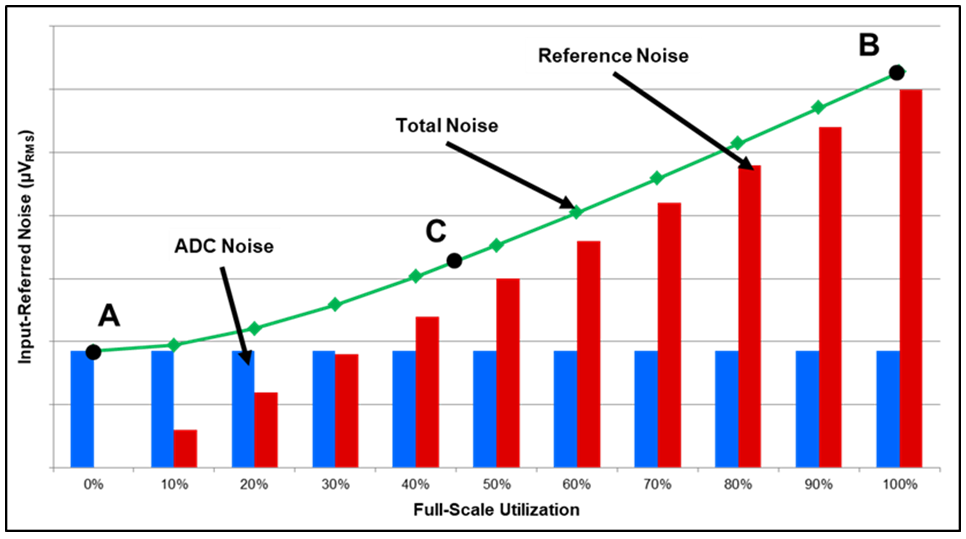If you have a related question, please click the "Ask a related question" button in the top right corner. The newly created question will be automatically linked to this question.

• Resolved

# [FAQ] ADC Noise: How do you calculate the amount of reference noise passing into your system?

How do you calculate the amount of reference noise passing into your system when using Delta-Sigma ADCs?

Regards,
Collin Wells

• One of the most interesting characteristics of reference noise is that it changes linearly with how much of the ADC’s FSR that you use. If you have a very small input signal, you won’t see much reference noise – and can potentially use a noisier reference as a result. Or if your input signals are greater than mid-scale, you can expect the reference noise to dominate. In this case, always make sure that the ADC noise and reference noise are comparable. Figure 7 qualitatively plots reference noise, ADC noise and total noise as a function of FSR utilization.Figure 7: Reference noise, ADC noise and total noise as a function of FSR utilization

What do the key points – A, B and C – on this plot represent? And how does changing your input signal vs. changing your system gain affect reference noise? Find the answers to these questions in part 8.

Regards,
Collin Wells